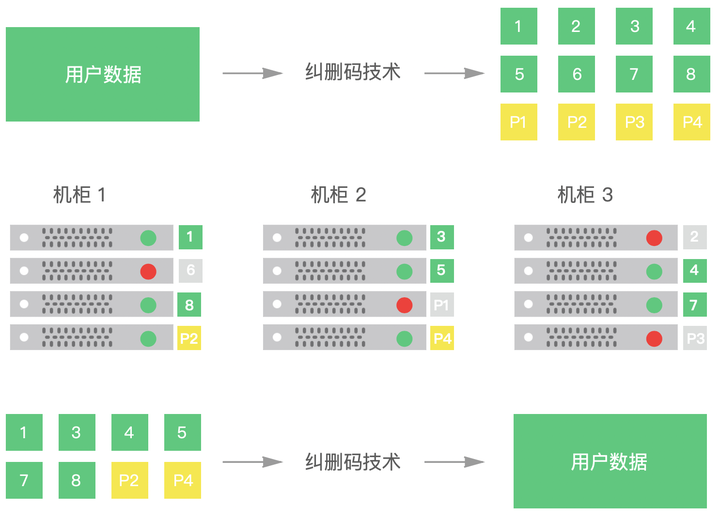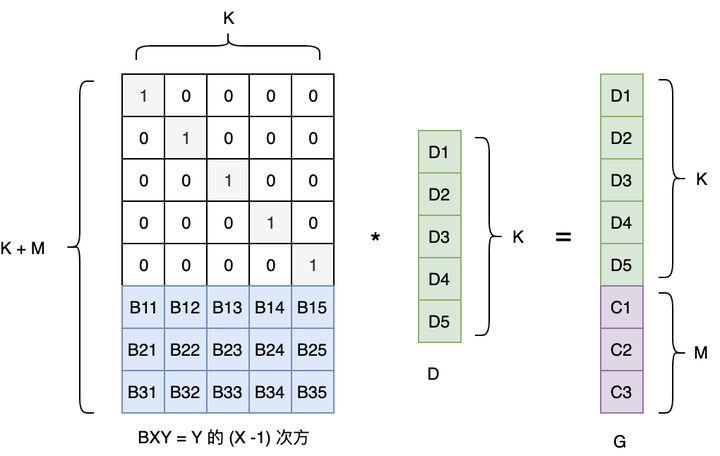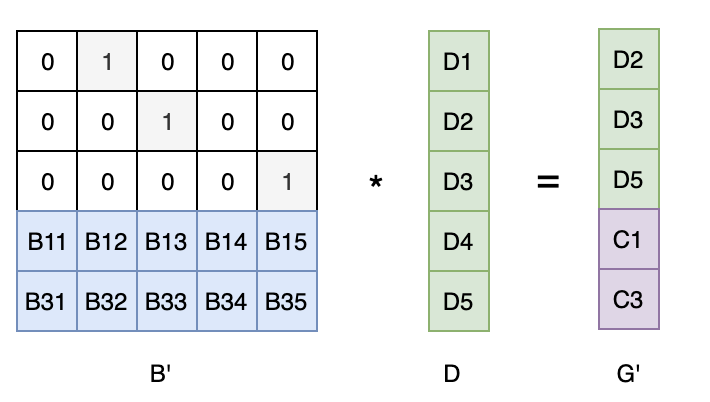FEC：Forward Error Correction，前向纠错

FEC 是一种通过在网络传输中增加数据包的冗余信息，使得接收端能够在网络发生丢包后利用这些冗余信息直接恢复出丢失的数据包的一种方法。

FEC 的基础理论：异或

```0 ^ 0 = 0
1 ^ 1 = 0
0 ^ 1 = 1
1 ^ 0 = 1```

```恒等律：X ^ 0 = X

XOR 的应用案例

```// 例如：求 10100001 中 1 的数量是奇数还是偶数
// 结果为 1 就是奇数个 1，结果为 0 就是偶数个 1
1 ^ 0 ^ 1 ^ 0 ^ 0 ^ 0 ^ 0 ^ 1 = 1```

```c = a ^ b
a = a ^ (b ^ b) = (a ^ b) ^ b = c ^ b
b = (a ^ a) ^ b = a ^ c```

```d = a ^ b ^ c
a = a ^ (b ^ b) ^ (c ^ c) = (b ^ c) ^ (a ^ b ^ c) = b ^ c ^ d
b = (a ^ a) ^ b ^ (c ^ c) = (a ^ c) ^ (a ^ b ^ c) = a ^ c ^ d
c = (a ^ a) ^ (b ^ b) ^ c = (a ^ b) ^ (a ^ b ^ c) = a ^ b ^ d```Reed-Solomon Codes

RS codes 编码过程1.  把 K 个有效数据组成一个单位向量 D

2.  生成一个变换矩阵 B：由一个 K 阶的单位矩阵 和一个 K * M 的范德蒙特 矩阵（Vandemode）组成

3.  两个矩阵相乘得到的矩阵 G，即包含了 M 个冗余的 FEC 数据

RS codes 解码过程1.  对矩阵 B 和 D，分别取没有丢失的行构成 B‘ 和 G’

2.  根据如下公式，即可计算恢复出有效数据向量 D

`B' x D = G'  ->>>  D = B' 的逆 x G'`